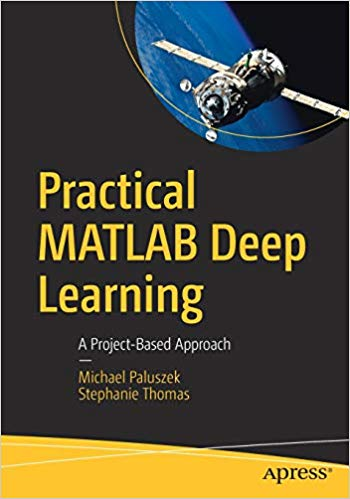# Practical MATLAB Deep Learning – pdf – 电子书免费下载Harness the power of MATLAB for deep-learning challenges. This book provides an introduction to deep learning and using MATLAB’s deep-learning toolboxes. You’ll see how these toolboxes provide the complete set of functions needed to implement all aspects of deep learning.

Along the way, you’ll learn to model complex systems, including the stock market, natural language, and angles-only orbit determination. You’ll cover dynamics and control, and integrate deep-learning algorithms and approaches using MATLAB. You’ll also apply deep learning to aircraft navigation using images.

Finally, you’ll carry out classification of ballet pirouettes using an inertial measurement unit to experiment with MATLAB’s hardware capabilities.

What You Will Learn

• Explore deep learning using MATLAB and compare it to algorithms
• Write a deep learning function in MATLAB and train it with examples
• Use MATLAB toolboxes related to deep learning
• Implement tokamak disruption prediction
Who This Book Is For

Engineers, data scientists, and students wanting a book rich in examples on deep learning using MATLAB.

ISBN:A Project-Based Approach

## 锦瑟无端五十弦，一弦一柱思华年

### 觉得文章有用就打赏一下文章作者

#### 支付宝扫一扫打赏#### 微信扫一扫打赏/* */